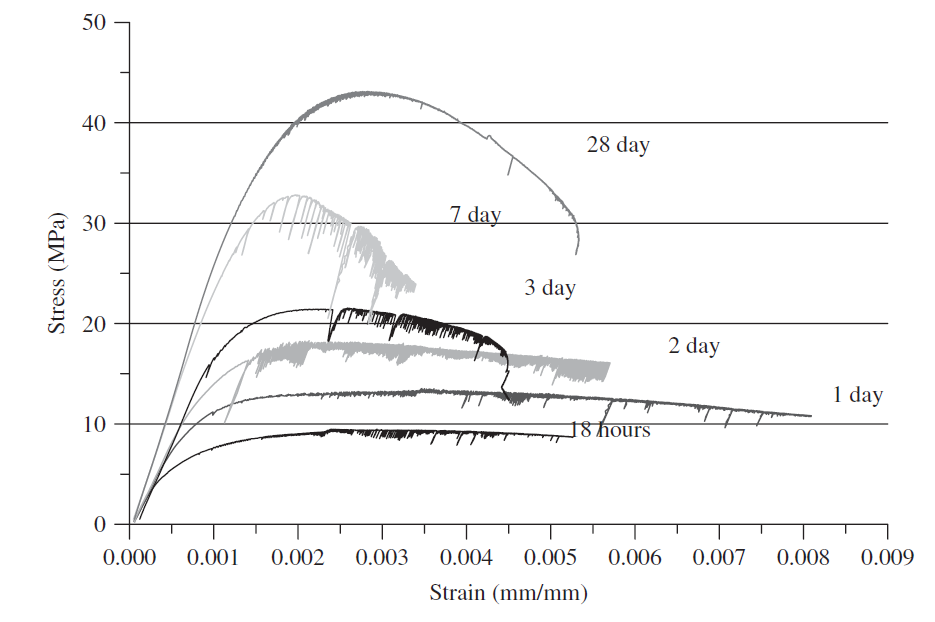## The concrete undergoes fast hydration for a period of 7 days from the day of casting, at 7 days concrete will gain almost 70% of it is strength, then the rate of hydration will decrease. At 28 days concrete will acquire nearly 95% of it is strength, then the hydration process will continue but at a slow rate. The properties of concrete at an early stage is different from mature concrete. The curves below showing concrete stress-strain curve for normal strength concrete(NSC) and high strength concrete(HSC) at different ages (18 hours, 1 day, 2 days... 7 days).

Figure 1 showing the stress-strain curve for NSC concrete, the curve consisting of ascending and descending part, it is noticed that the curve ascending part steeply go up after 7 days for NSC and after 2 days for HSC, the same for dropping element, with the increase of time both parts of curve become steeper. This means that the concrete is becoming more brittle. Brittle means concrete will not undergo high strain for a given high stress, at early age concrete was ductile. We can notice this from the curve shape, for low stress the strain is high, modulus of elasticity is the slope of the curve, secant modulus of elasticity is the slope of the line drawn from the origin of the curve to the point of 40% of ultimate strength of concrete. The secant modulus of elasticity of concrete increased with increasing of time. This means concrete become more stiff and brittle. It can be noticed that the concrete behave more ductile at an early stage, this will decrease with increasing of time and the curves below showing the decrease of strain for higher stress as the time increased.Figure 1(Stress-strain curve for NSC)Figure 2(stress-strain curve HSC)